# 把Excel表格通过MySql Workbench导入数据库表中的使用总结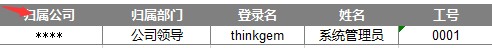（1）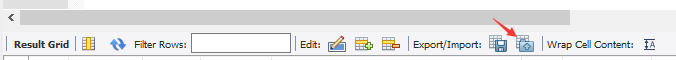（2）第二种方式较为复杂，但是可以实现手动对应：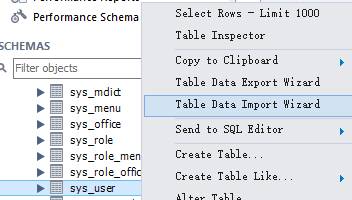–>next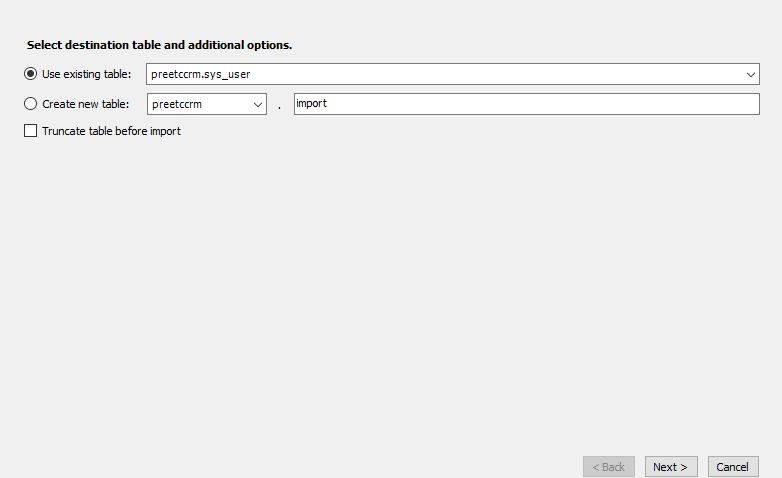–>next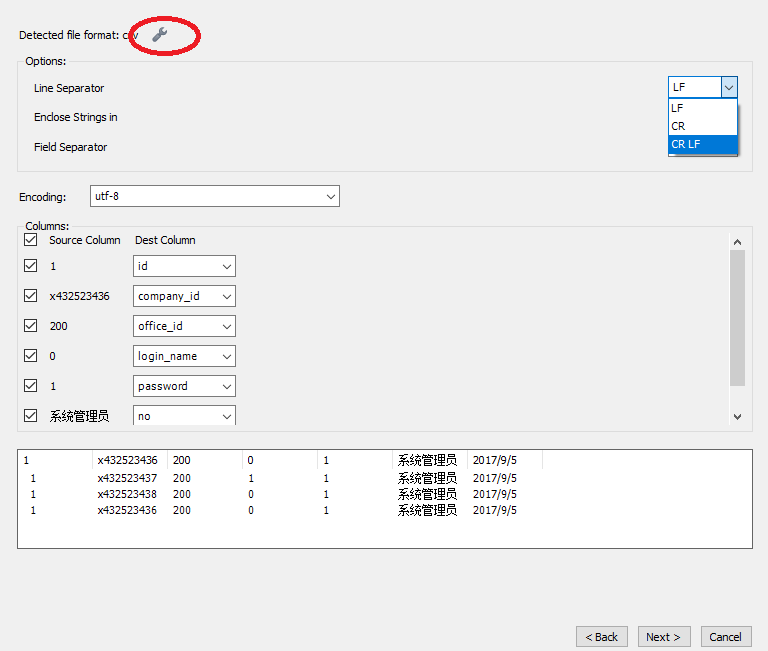‘按alt+f11进入VBE编辑窗口，然后在工程窗口插入一个模块
‘把下面的代码复制进去–保存，再次按alt+f11返回excel窗口
‘这时，按alt+f8打开宏窗口，运行randnum宏，如果你选中的是
‘一个单元格，就会产生32位 ABCDEF跟0123456789 的随机组合字符串
‘（代码亲测，希望对你有用）

 Sub randnum() Dim j, p As Integer, tem, x(16) As String x(1) = "0" x(2) = "1" x(3) = "2" x(4) = "3" x(5) = "4" x(6) = "5" x(7) = "6" x(8) = "7" x(9) = "8" x(10) = "9" x(11) = "A" x(12) = "B" x(13) = "C" x(14) = "D" x(15) = "E" x(16) = "F" For j = 1 To 32 Randomize p = Int(16 * Rnd) + 1 tem = tem & x(p) Next j If Selection.Count = 1 Then Selection.Value = tem End If End Sub 
Rnd 函数返回小于 1 但大于或等于 0 的值；‘&’表示连接字符串，要注意前后加个空格，不能像公式那样前后紧贴在一起，否则有的时候会报错。

Load Data InFile ‘D:/import.txt’ Into Table “ ####”lines terminated by ‘\r\n’;

Error Code: 1290. The MySQL server is running with the –secure-file-priv option so it cannot execute this statement

1.进入mysql查看secure_file_prive的值
\$mysql -u root -p
mysql>SHOW VARIABLES LIKE “secure_file_priv”;
secure_file_prive=null – 限制mysqld 不允许导入导出
secure_file_priv=/tmp/ – 限制mysqld的导入导出只能发生在/tmp/目录下
secure_file_priv=’ ’ – 不对mysqld 的导入 导出做限制

2、在目录C:\ProgramData\MySQL\MySQL Server 5.7下找到my.ini文件，然后修改 secure_file_prive为’ ‘,或者把导入文件放入指定的文件夹，即可完成导入；